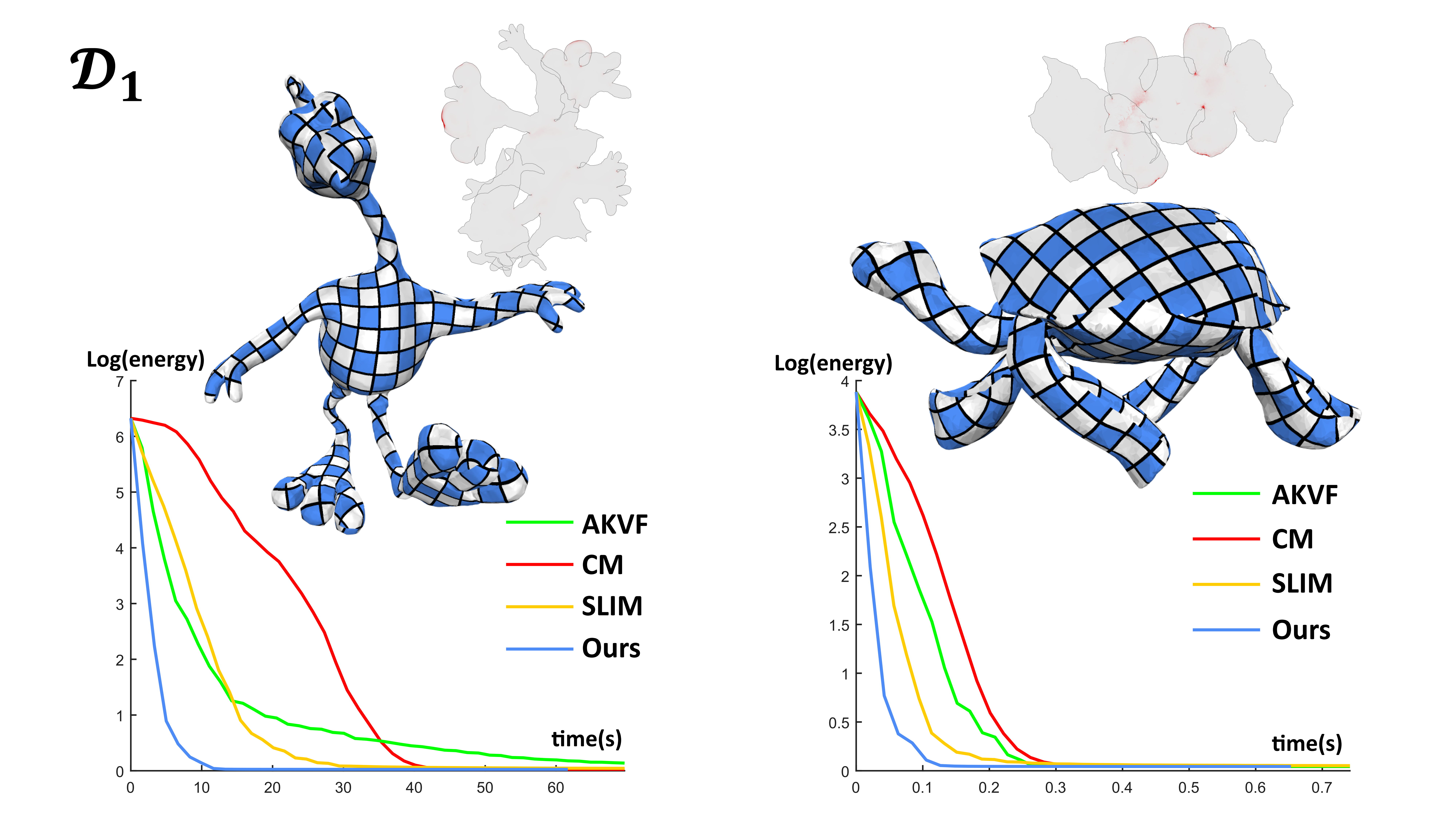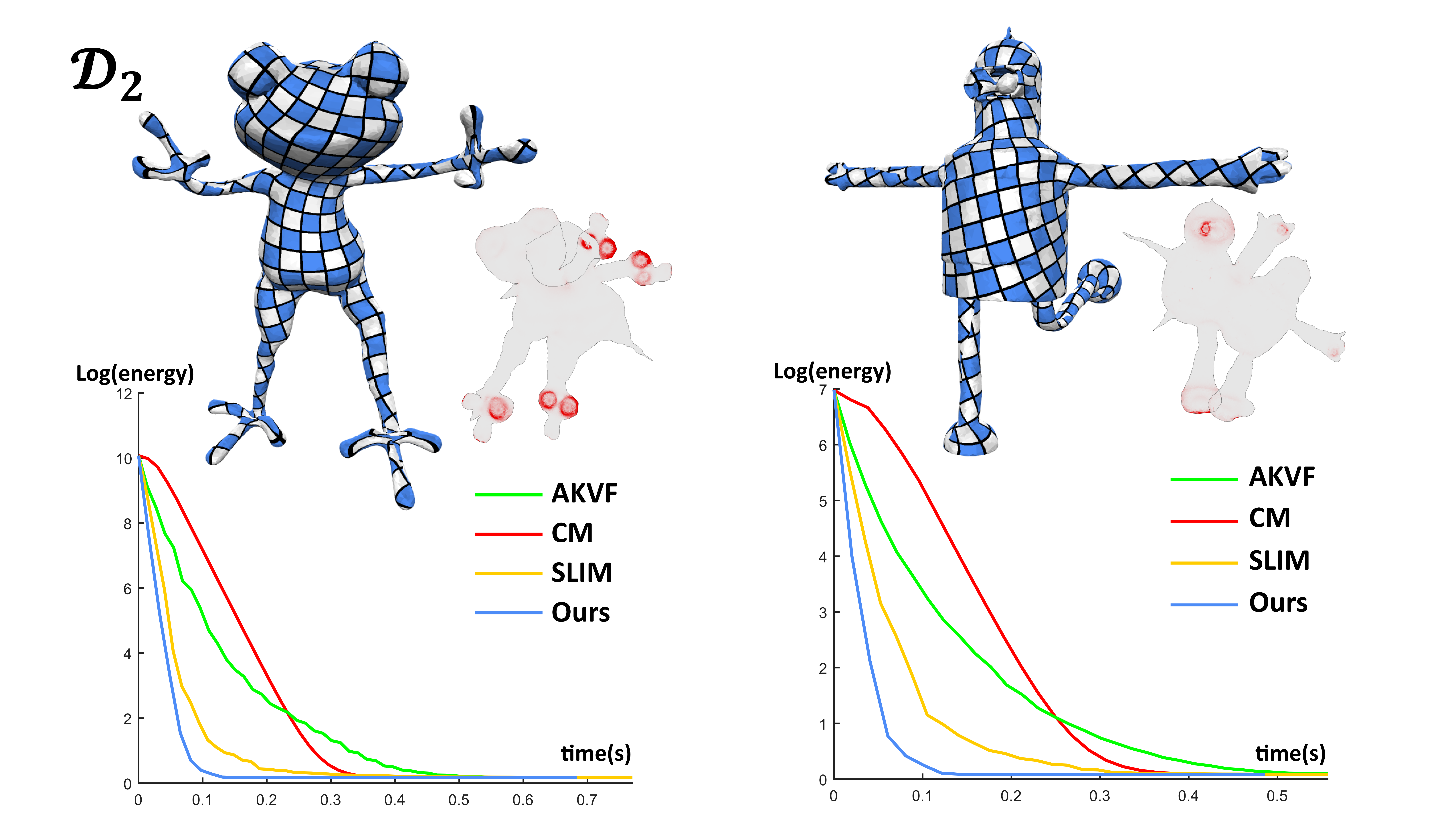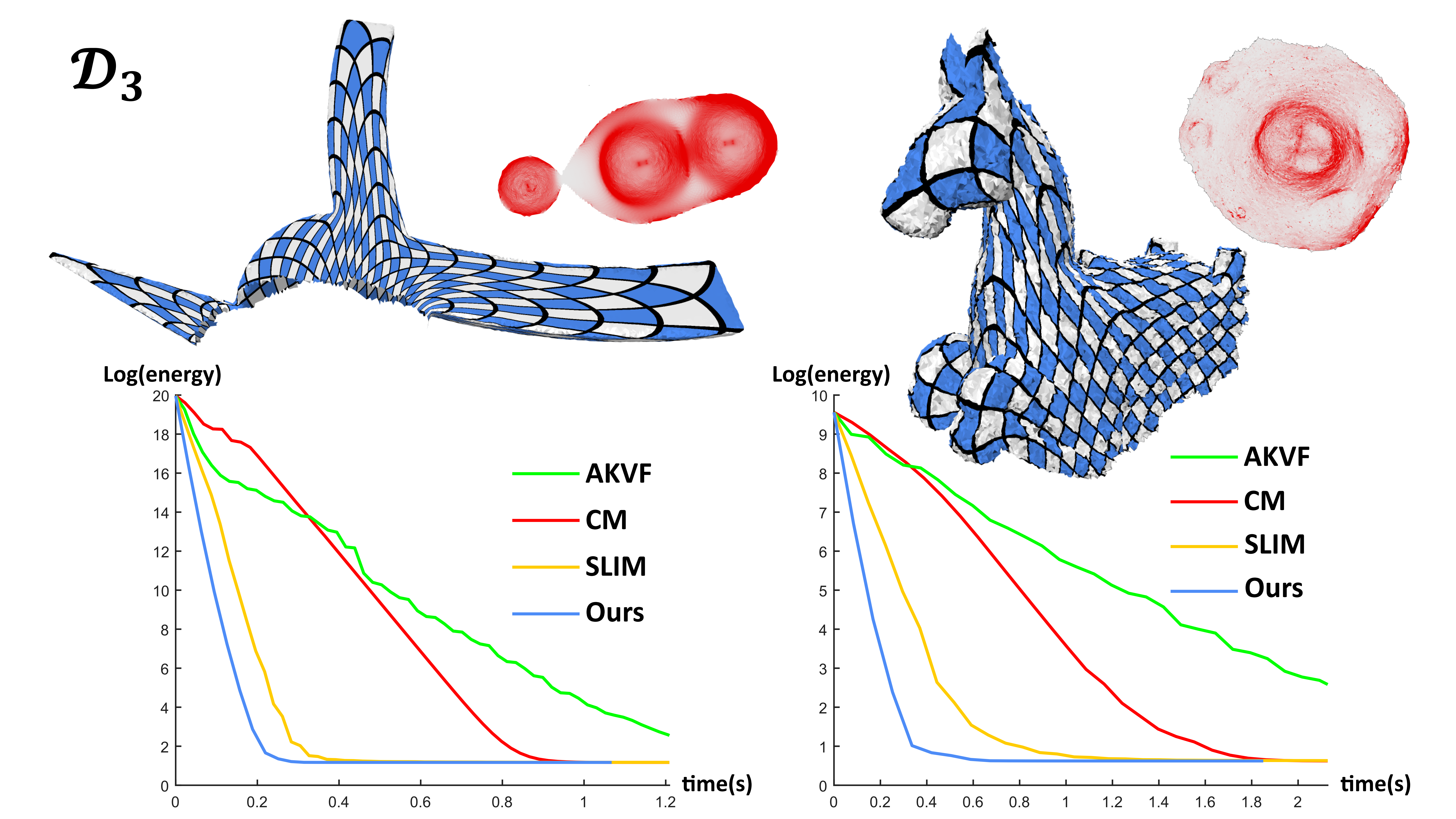Data Set for Parameterizations

This large testing benchmark contains 20712 meshes. It starts from well cut meshes $$D_1$$, continues with moderately bad ones $$D_2$$, and ends with extremely challenging examples $$D_3$$. If you use our data set, please cite  and .

$$D_1$$ (Download)Figure 1: Gallery of some selected models from $$D_1$$, which containts 10273 models. Since nearly half the models are very large, we use a program to subdivide the small sized meshes. Here we only provide the small sized meshes which are cut by our sphere-based method . If you want to test your parameterization method on these large-scale models, please download the code to generate them.Figure 2: Efficiency comparisons using two models from $$D_1$$. We select the scalable locally injective maps (SLIM) [Rabinovich et al. 2017], the approximate Killing vector field method (AKVF) [Claici et al. 2017], and the composite majorization method (CM) [Shtengel et al. 2017] as the competitors.

$$D_2$$ (Download)Figure 3: Gallery of some selected models from $$D_2$$, which containts 6189 models.Figure 4: Efficiency comparisons using two models from $$D_2$$.

$$D_3$$ (Download)Figure 5: Gallery of some selected models from $$D_3$$, which containts 4250 models.Figure 6: Efficiency comparisons using two models from $$D_3$$.

Reference

: Ligang Liu, Chunyang Ye, Ruiqi Ni, Xiao-Ming Fu. Progressive Parameterizations. ACM Transactions on Graphics (SIGGRAPH) 37(4), 2018. [Bibtex].
: Shuangming Chai, Xiao-Ming Fu, Xin Hu, Yang Yang, Ligang Liu. Sphere-based Cut Construction for Planar Parameterizations. Computer & Graphics (SMI 2018). [Bibtex].